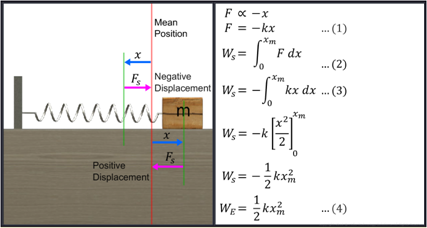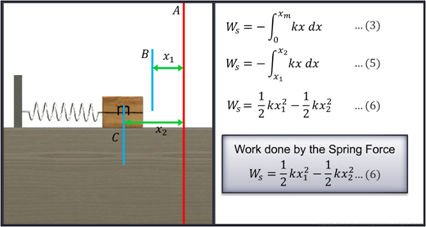Notes On Potential Energy of a Spring - CBSE Class 11 Physics
 Consider a block attached to a spring placed on a smooth, horizontal surface. The other end of the spring is attached to a rigid wall. The position at which the spring is at rest initially is considered to be the ‘mean position’ with no displacement of the spring. Then, the spring is compressed and released. You can observe the spring oscillating to and fro from the rest or mean position. The displacement of the block is always measured from the mean or rest position. The spring here is under the action of a variable force, known as the spring force, which always acts towards the mean position or in the opposite direction to the displacement. When the spring is stretched, the displacement is positive. When the spring is compressed, the displacement is negative. The spring force is the restoring force which is responsible for bringing the block attached to the spring back to its mean position.                                                The spring force (F), is directly proportional to the displacement (x)’ from the mean position. Thus, we see that the spring force ‘F ax’. We say -x as the spring force is always directed opposite to the direction of displacement or spring force ‘,where k is the spring constant.   The above Equation represents the force law for the spring known as Hooke’s Law.Work done ‘W ‘by the spring force is calculated as shown in the figures.F d x in the limits 0 to x m, where x m is the maximum displacement of the spring. According to the Work-Energy Theorem, the work done by a net force on an object is equal to the change in its kinetic energy.

#### Summary

 Consider a block attached to a spring placed on a smooth, horizontal surface. The other end of the spring is attached to a rigid wall. The position at which the spring is at rest initially is considered to be the ‘mean position’ with no displacement of the spring. Then, the spring is compressed and released. You can observe the spring oscillating to and fro from the rest or mean position. The displacement of the block is always measured from the mean or rest position. The spring here is under the action of a variable force, known as the spring force, which always acts towards the mean position or in the opposite direction to the displacement. When the spring is stretched, the displacement is positive. When the spring is compressed, the displacement is negative. The spring force is the restoring force which is responsible for bringing the block attached to the spring back to its mean position.                                                The spring force (F), is directly proportional to the displacement (x)’ from the mean position. Thus, we see that the spring force ‘F ax’. We say -x as the spring force is always directed opposite to the direction of displacement or spring force ‘,where k is the spring constant.   The above Equation represents the force law for the spring known as Hooke’s Law.Work done ‘W ‘by the spring force is calculated as shown in the figures.F d x in the limits 0 to x m, where x m is the maximum displacement of the spring. According to the Work-Energy Theorem, the work done by a net force on an object is equal to the change in its kinetic energy.

Previous
Next